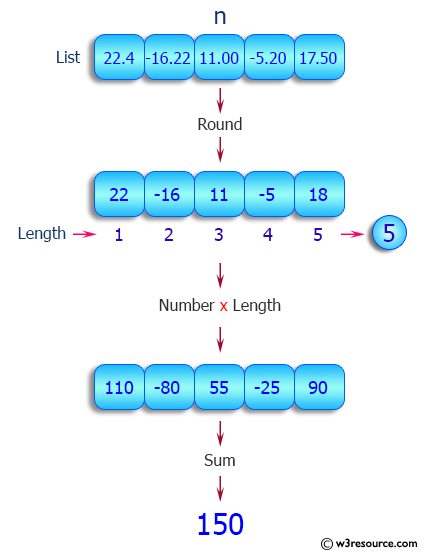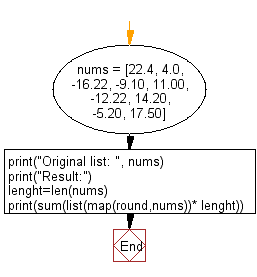﻿ Python: Round every number of a given list of numbers and print the total sum multiplied by the length of the list - w3resource# Python: Round every number of a given list of numbers and print the total sum multiplied by the length of the list

## Python List: Exercise - 83 with Solution

Write a Python program to round every number of a given list of numbers and print the total sum multiplied by the length of the list.

Sample Solution:

Python Code:

``````nums = [22.4, 4.0, -16.22, -9.10, 11.00, -12.22, 14.20, -5.20, 17.50]
print("Original list: ", nums)
print("Result:")
lenght=len(nums)
print(sum(list(map(round,nums))* lenght))
```
```

Sample Output:

```Original list:  [22.4, 4.0, -16.22, -9.1, 11.0, -12.22, 14.2, -5.2, 17.5]
Result:
243
```

Pictorial Presentation:Flowchart:## Visualize Python code execution:

The following tool visualize what the computer is doing step-by-step as it executes the said program:

Python Code Editor:

Have another way to solve this solution? Contribute your code (and comments) through Disqus.

What is the difficulty level of this exercise?

Test your Python skills with w3resource's quiz

﻿

## Python: Tips of the Day

Floor Division:

When we speak of division we normally mean (/) float division operator, this will give a precise result in float format with decimals.

For a rounded integer result there is (//) floor division operator in Python. Floor division will only give integer results that are round numbers.

```print(1000 // 300)
print(1000 / 300)```

Output:

```3
3.3333333333333335```